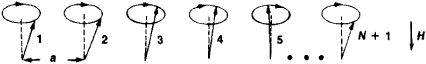# Spin Wave

Also found in: Dictionary, Wikipedia.
Related to Spin Wave: Magnon

## spin wave

[′spin ‚wāv]
(solid-state physics)
A sinusoidal variation, propagating through a crystal lattice, of that angular momentum which is associated with magnetism (mostly spin angular momentum of the electrons).

## Spin Wave

(1) In magnetically ordered media, spin waves are waves of disturbances of the spin ordering. In ferromagnetic materials, antiferromagnetic materials, and ferrites, the spins of atoms and the magnetic moments associated with the spins are strictly ordered in the ground state. Because of the strong exchange interaction between atoms, the departure of the magnetic moment of any atom from its equilibrium position is not localized but propagates through the medium in the form of a wave. Spin waves are an elementary motion of the magnetic moments in magnetic media. The existence of spin waves was predicted by F. Bloch in 1930.

A spin wave, like any wave, is characterized by the dependence of the frequency ѡ on the wave vector k (the dispersion relation). Several types of spin waves can exist in complex magnetic media (crystals with several magnetic sublattices); the dispersion relation of such spin waves depends essentially on the magnetic structure of the substance.

Spin waves can be given a pictorial classical interpretation by considering a chain of N atoms in a magnetic field H where the spacing between adjacent atoms is a (see Figure 1). If the wave vector of the spin wave is k = 0, then all the spins are precessing in phase about the direction of the field H. The frequency of this homogeneous precession equals the Larmor frequency ѡ0. For k ≠ 0, the spins execute inhomogeneous precession: the precessions of the separate spins (1, 2, 3, and so on) are not in phase, and the phase shift between neighboring atoms is equal to ka (see Figure 1). The frequency ω(k) of inhomogeneous precession is greater than the frequency of homogeneous precession ω0. If the forces of interaction between the spins are known, the function ta(k) can be calculated.Figure 1. Precession of W spin vectors in a linear chain of atoms (instantaneous “picture”)

For long spin waves (ka << 1) in ferromagnetic materials, this function is simple:

ω(k) = ω0 + ωe (ak)2

The quantity ħωe is of the order of magnitude of the exchange integral between neighboring atoms. As a rule, ωe >> ω0. The frequency of homogeneous precession ω0 is determined by the crystal anisotropy and the magnetic field H applied to the crystal: ѡ0= g(βM + H), where g is the gyromagnetic ratio, β is the anisotropy constant, and M is the magnetization at T = 0°K. From a quantum-mechanical consideration of a system of interacting spins it is possible to calculate the dispersion relation of the spin waves for different crystal lattices for an arbitrary ratio of the length of the spin wave and the lattice constant of the crystal.

Spin waves are regarded as corresponding to a quasi-particle called a magnon. At T = 0°K, there are no magnons in magnetic media. At higher temperatures, magnons appear, the number increasing with increasing temperature. The number of magnons in ferromagnetic materials is approximately proportional to T312; in antiferromagnetic materials, the number is approximately proportional to T3. An increase in the number of magnons leads to a decrease in the magnetic ordering. Thus, because of the increase in the number of spin waves with increasing temperature, there is a decrease in the magnetization of a ferromagnetic material; according to Bloch’s law, the change in magnetization is ΔMT) ~ T312.

Spin waves are manifested in the thermal, high-frequency, and other properties of magnetic media. When neutrons are inelasti-cally scattered by magnetic media, spin waves are excited in the media. Scattering of neutrons is one of the most effective methods for experimental determination of the dispersion relations of spin waves (seeNEUTRON DIFFRACTION ANALYSIS).

(2) In nonmagnetic metals, spin waves are oscillations in the spin density of the conduction electrons caused by the exchange interaction of the electrons. The existence of spin waves in nonmagnetic metals is manifested in certain features of electron paramagnetic resonance (EPR), in particular, the ability of metal plates to transmit selectively electromagnetic waves with frequencies close to the EPR frequency.

### REFERENCES

Akhiezer, A. I., V. G. Bar’iakhtar, and S. V. Peletminskii. Spinovyevolny. Moscow, 1967.

M. I. KAGANOV

References in periodicals archive ?
To overcome the trade-off between current density and the skyrmion velocities, the solution based on the spin Seebeck effect [13, 14] and spin wave [15, 16] (SW) are proposed recent years.
The researchers built a prototype eight-terminal device consisting of a magnetic matrix with micro-antennae placed on the periphery of the matrix to excite and detect spin waves. The principle of operation is based on the effect of spin wave interference, which is similar to the operation of optical holographic devices.
In this paper, contributions of the electron thermal movement, spin wave, and lattice thermal movement in different magnetization filed can be investigated by measuring the [Pr.sub.0.65][Ca.sub.0.35]Mn[O.sub.3] low temperature specific heat.
For finite wave-vector spin waves a dispersion of the spin wave frequencies with the wave-vector can be found.
The collective excitations in quantum spin systems can broadly be divided into two categories: (i) conventional ones, namely, spin waves (quantised as magnons) and (ii) topological ones, namely, vortices and merons.
At low temperatures, the spin wave excitations are dominated by the uniform mode (q = 0 spin waves), resulting in a linear temperature dependence of the magnetization in contrast to the Bloch T3/2 law valid for bulk materials .
"Our idea was to use such spin wave interactions for generating efficient spin currents," Kurebayashi explained.
NIST researchers have imaged the spatial structure of a standing spin wave for the first time in a dilute gas.
Unlike the conventional way, the information can be sent in the form of a "spin wave" that travels through the cluster of electrons in a conductor.

Site: Follow: Share:
Open / Close